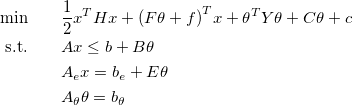Main /

# DemosOnOptimizationInterface

## Demos on Optimization Interface

The Multi-Parametric Toolbox supports various solvers for solving optimization problems such as linear programs (LP), quadratic programs (QP), mixed-integer linear problems (MILP), mixed-integer quadratic problems (MIQP), linear-complementarity problems (LCP), and their parametric extensions. The unified gateway for formulating optimization problems represents the class Opt.

The concept of solving optimization problems in MPT follows two steps:

1. Formulate an optimization problem using Opt class.
2. Call a method solve to get a solution.

When formulating the optimization problem, user is supposed to provide the problem data to the Opt class that accepts the following formatorBased on the problem data, the Opt class determines the type of the problem and attaches an appropriate solver from the list of installed solvers. The solvers are sorted according to a priority list that can be changed.

For more details on formulating and solving optimization problems, inspect

mpt_demo_opt1

Continue reading by exploring Demos on Formulation of Control Problems.